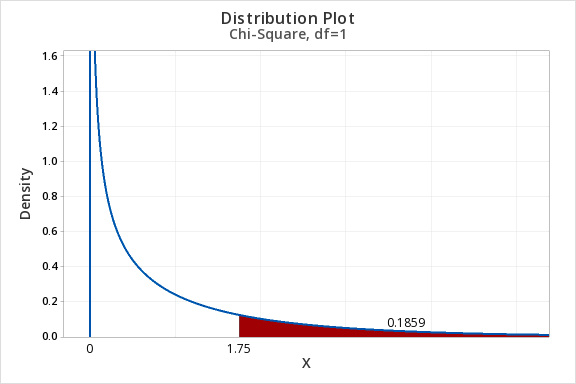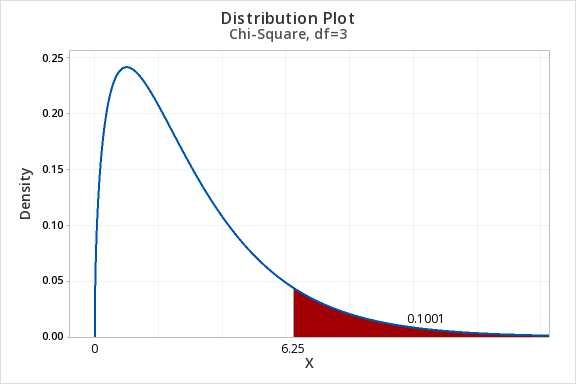# 11.1.3 - Probability Distribution Plots

11.1.3 - Probability Distribution Plots

In previous lessons you have constructed probabilities distribution plots for normal distributions, binomial distributions, and $$t$$ distributions. This week you will use the same procedure to construct a probability distribution plot for the chi-square distribution.

## Minitab® – Constructing a Probability Distribution Plot

Chi-square tests of independence are always right-tailed tests. Let's find the area of a chi-square distribution with 1 degree of freedom to the right of $$\chi^2 = 1.75$$. In other words, we're looking up the $$p$$ value associated with a chi-square test statistic of 1.75.

1. In Minitab, select Graph > Probability Distribution Plot > View Probability
2. Choose Chi-Square for the Distribution
3. For Distribution select Chi-Square
4. For Degrees of freedom enter 1
5. Select A specified X value
6. Select Right tail
7. For X value enter 1.75
8. Select OK and OK

This should result in the following output:## Example: Area to the Right of Chi-Sq = 6.25, df=3

Construct a chi-square distribution with 3 degrees of freedom to find the area to the right of a chi-square value of 6.25.

1. In Minitab, select Graph > Probability Distribution Plot > View Probability
2. Choose Chi-Square for the Distribution
3. For Distribution select Chi-Square
4. For Degrees of freedom enter 3
5. Select A specified X value
6. Select Right tail
7. For X value enter 6.25
8. Select OK and OKThe area to the right of 6.25 in the chi-square distribution with 3 degrees of freedom is 0.1001.

  Link ↥ Has Tooltip/Popover Toggleable Visibility